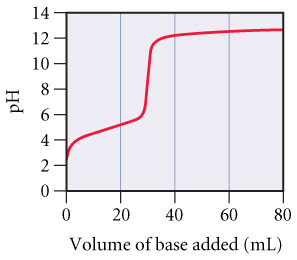# Problem: Consider the following curve for the titration of a weak monoprotic acid with a strong base and answer each of the following questions.At what volume of added base does pH = pKa?

89% (95 ratings)
###### Problem Details

Consider the following curve for the titration of a weak monoprotic acid with a strong base and answer each of the following questions.At what volume of added base does pH = pKa?